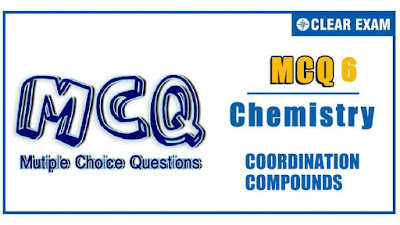## [LATEST]\$type=sticky\$show=home\$rm=0\$va=0\$count=4\$va=0

JEE Advanced is a high level exam that checks your concept by putting various types of questions. The questions are in a different format from JEE mains, herein the questions are : single correct multiple choice questions, multiple correct multiple choice questions, statement based questions and comprehension type questions..

Q1.
Aqueous Fe (III) ion develops intense red colour with SCN^-while Fe(II) does not. It is due to
•  Fe (III) ion forms a chare transfer complex with transfer complex with SCN^- ions
•  Fe(III) is reduced to Fe(I) which is deep red in colour
•  SCN^- ion oxidizes to CN^- ion that forms red complex with Fe (III) ion
•  SCN^- ion does not form any complex with Fe (II) ion
Solution
(c)

Q2.
Select the incorrect statement
•  [Ni(en)_3 ]^(2+) is less stable than [Ni(NH_3 )_6 ]^(2+)
•  Increase in stability of the complexes due to presence of multidentate cyclic ligand is called macrocyclic effect
•  A complex ion that exchanges ligands slowly is said to be non-labile or inert
•  For a given ion and ligand, greater the charge on the metal ion, greater the stability
Solution
(a)Chelate complex thus more stable than [Ni(NH_3 )_6 ]^(2+)

Q3. Why is [Ni(en)_3 ]^(2+) nearly10^10 times more stable than [Ni(NH_3 )_6 ]^(2+)
•  NH_3evaporates easily and causes instability to[Ni(NH_3 )_6 ]^(2+) complex
•  Six NH_3 ligands cause steric hindrance around the Ni^(2+)centre
•  ‘en’ is a chelating ligand and forms thermodynamically more stable complexes
•  NH_3is the weakest ligand known
Solution
(c)

Q4. The complex [Co(NH_3 )_5 (NO_2 )]^(2+)and [Co(N〖H_3)〗_5 (ONO)]^(2+) are called
•  Ionization isomer
•  Coordination isomer
•  Geometrical isomer
Solution
(b)
Ligands are

Q5.Ink contains Fe^(3+).Spot of ink can be removed by addition of C_2 O_4^(2-), Complex formed is
•  [Fe(C_2 O_4 )_6 ]^(3-)
•  [Fe(C_2 O_4 )_3 ]^(3-)
•  [Fe(C_2 O_4 )_3 ]^(2-)>
•  [Fe(C_2 O_4 )_3 ]^(4-)
Solution
(b)

•  Is the molecular complex
•  Has four ions in the aqueous solution
• Primary valency of cobalt is six
•  All the above are correct
Solution
(a) [Co(NH_3 )_3 Cl_3]⇌ no ionization Thus, is the molecular complex

Q7.Lead poisoning in the body can be removed by
•  EDTA in the form of calcium dihydrogen salt
•  cis-platin
•  Zeise’s salt
•  DMG
Solution
(a) EDTA (in the form of dihydrogen salt) forms insoluble compound with Pb^(2+)and is thus drained out

Q8.What is CFSE of a free Co(II) ion on forming the tetrahedral chloro complex [CoCl_4 ]^(2-) (in the units of ∆_0)
•  0.6
•  1.2
•  1.8
•  2.4
Solution
(c)

Q9.Potassium trisoxalatochromate (III) is
•  K_3 [Cr(C_2 O_4 )_3]
•  K[Cr(C_2 O_4 )_3]
•  K_2 [Cr(C_2 O_4 )_3]
•  K_4 [Cr(C_2 O_4 )_3]
Solution
(a) Cr^(3+)+3(C_2 O_4 )^(2-)→K^+ [Cr(C_2 O_4 )_3 ]^(3-) Thus, complex is K_3 [Cr(C_3 O_4 )_3]

Q10. Which of the following complexes exhibit optical isomerism?
•  Trans-tetramminedithiocyanatochromium (III) ion
•  Cis-diamminedicarbonatocobaltate (III) ion
•  Trans-diamminedicarbonatocobaltate (III) ion
• Cis-glycinatoplatinum (II)
Solution
(b)Mirror –images are not superimposable#### Written by: AUTHORNAME

AUTHORDESCRIPTION## Want to know more

Please fill in the details below:

## Latest NEET Articles\$type=three\$c=3\$author=hide\$comment=hide\$rm=hide\$date=hide\$snippet=hide

Name

ltr
item
BEST NEET COACHING CENTER | BEST IIT JEE COACHING INSTITUTE | BEST NEET & IIT JEE COACHING: Coordination Compounds - Quiz 6
Coordination Compounds - Quiz 6